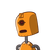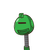# In ∆XYZ , X Y = 90° YZX= 30 and YXZ=60°seg XY = 4 cmthe find seg XZ​

In ∆XYZ , X Y = 90° YZX= 30 and YXZ=60°
seg XY = 4 cm
the find seg XZ​

### 2 thoughts on “In ∆XYZ , X Y = 90° YZX= 30 and YXZ=60°<br />seg XY = 4 cm<br />the find seg XZ​”

1.XZ=8CM

Step-by-step explanation:

as per the given information it is prove that the above Triangle is 30°60° 90° Triangle

by 30-60-90 theorem,

s

side \: opposite \: to \: 30 = \frac{1}{2} \times hypotenusesideoppositeto30=

2

1

×hypotenuse

XY=½XZ

XZ=2×XY

XZ=2×4

XZ=8 cm

2.In right angled triangle yxz (angle x=90°) :- (xy)^2+(xz)^2= (yz)^2.

or. (9)^2+(xz)^2=(12)^2.

or. (xz)^2= 144–81=63.

Or. xz = 3√7 cm.

Area of right angled triangle yxz= 1/2. (xy)×(xz)………………….(1)

Also area of right angled triangle yxz = 1/2.(ox)×(yz)………………………(2).

from eqn. (1) and (2)

1/2.(ox)×(yz) =1/2.(xy)×(xz).

or. ox = (xy)×(xz)/(yz). = (9×3√7)/(12) = 9√7/4= 5.95 cms. Answer.